# Local Maxima And Minima

Maxima and Minima are one of the most common concepts in differential calculus. A branch of Mathematics called “Calculus of Variations” deals with the maxima and the minima of the functional. The calculus of variations is concerned with the variations in the functional, in which small change in the function leads to the change in the functional value.

The first variation is defined as the linear part of the change in the functional, and the second part of the variation is defined in the quadratic part. Functional is expressed as the definite integrals which involve the functions and their derivatives.

The functions that maximize or minimize the functional are can be found using the Euler – Lagrange of the calculus of variations. These two Latin maxima and minima words basically mean the maximum and minimum value of a function respectively, which is quite evident. The maxima and minima are collectively called “Extrema”. Here, we assume our function to be continuous for its entire domain. Before knowing how to find maxima and minima, we should first learn about derivatives. Assuming that you all know how to find derivatives, let us go ahead and learn about some curves. What are the curves?

Also, learn:

## What are the Curves?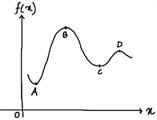Figure 1: Curves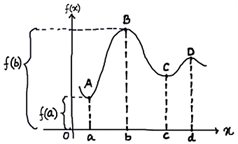Figure 2: Value of a Function

A curve is defined as one-dimensional continuum. In figure 1, that curve is graph of a function

$$\begin{array}{l} f ~in~ x \end{array}$$
.
$$\begin{array}{l} f(x) \end{array}$$
represents the value of function at
$$\begin{array}{l} x \end{array}$$
. The value of
$$\begin{array}{l} f \end{array}$$
when
$$\begin{array}{l} x = a \end{array}$$
, will be
$$\begin{array}{l} f(a) \end{array}$$
. Similarly, for
$$\begin{array}{l} B,~ C ~and ~D\end{array}$$
. You can refer fig. 2 to understand this. From the figure it is quite clear that the value of the given function has its maximum value at x=b, i.e.
$$\begin{array}{l} f(b) \end{array}$$
.

Interval of a function plays a very important role to find extreme values of a function. If the interval for which the function

$$\begin{array}{l} f \end{array}$$
is defined in
$$\begin{array}{l} R \end{array}$$
, then we can’t talk about maxima and minima of
$$\begin{array}{l} f \end{array}$$
. We can understand it logically that though
$$\begin{array}{l} f(b) \end{array}$$
appears to have the maximum value, we can’t be sure it has the largest value till we have seen the graph for its entire domain.

## Local Maxima and Minima

We may not be able to tell whether

$$\begin{array}{l} f(b) \end{array}$$
is the maximum value of
$$\begin{array}{l} f \end{array}$$
, but we can give some credit to point . We can do this by declaring
$$\begin{array}{l} B \end{array}$$
as the local maximum for function
$$\begin{array}{l} f \end{array}$$
. These are also called relative maxima and minima. These local maxima and minima are defined as:

• If
$$\begin{array}{l} f(a) \leq f(x) \end{array}$$
for all
$$\begin{array}{l} x \end{array}$$
in
$$\begin{array}{l} P’s\end{array}$$
neighborhood (within the distance nearby
$$\begin{array}{l} P \end{array}$$
, where
$$\begin{array}{l} x = a \end{array}$$
),
$$\begin{array}{l} f \end{array}$$
is said to have a local minimum at
$$\begin{array}{l} x = a \end{array}$$
.
• If
$$\begin{array}{l} f(a) \geq f(x) \end{array}$$
for all in
$$\begin{array}{l} P’s \end{array}$$
neighborhood (within the distance nearby
$$\begin{array}{l} P \end{array}$$
, where
$$\begin{array}{l} x = a \end{array}$$
),
$$\begin{array}{l} f \end{array}$$
is said to have a local maximum at
$$\begin{array}{l} x = a \end{array}$$
.

In the above example,

$$\begin{array}{l} B ~and~ D \end{array}$$
are local maxima and
$$\begin{array}{l} A ~and~ C\end{array}$$
are local minima. Local maxima and minima are together referred to as Local extreme.

Let us now take a point

$$\begin{array}{l} P \end{array}$$
, where
$$\begin{array}{l} x = a\end{array}$$
and try to analyze the nature of the derivatives. There are total of four possibilities:

• If
$$\begin{array}{l} f'(a) = 0 \end{array}$$
, the tangent drawn is parallel to
$$\begin{array}{l} x -axis \end{array}$$
, i.e. slope is zero. There are three possible cases:
• The value of
$$\begin{array}{l} f \end{array}$$
, when compared to the value of
$$\begin{array}{l} f \end{array}$$
at
$$\begin{array}{l} P \end{array}$$
, increases if you move towards right or left of
$$\begin{array}{l} P \end{array}$$
(Local minima: look like valleys)
• The value of
$$\begin{array}{l} f \end{array}$$
, when compared to the value of
$$\begin{array}{l} f \end{array}$$
at
$$\begin{array}{l} P \end{array}$$
, decreases if you move towards right or left of
$$\begin{array}{l} P \end{array}$$
(Local maxima: look like hills)
• The value of
$$\begin{array}{l} f \end{array}$$
, when compared to the value of
$$\begin{array}{l} f \end{array}$$
at
$$\begin{array}{l} P \end{array}$$
, increases and decreases as you move towards left and right respectively of
$$\begin{array}{l} P \end{array}$$
(Neither: looks like a flat land)
• If, the tangent is drawn at a negative slope. The value of f'(a), at p, increases if you move towards left of and decreases if you move towards the right of . So, in this case, also, we can’t find any local extrema.
• If, the tangent is drawn at a positive slope. The value of f'(a), at P, increases if you move towards the right of and decreases if you move towards left of . So, in this case, we can’t find any local extrema.
• $$\begin{array}{l} f’ \end{array}$$
doesn’t exist at point
$$\begin{array}{l} P \end{array}$$
, i.e. the function is not differentiable at
$$\begin{array}{l} P \end{array}$$
. This normally happens when the graph of
$$\begin{array}{l} f \end{array}$$
has a sharp corner somewhere. All the three cases discussed in the previous point also hold true for this point.

To remember this, you can refer the Table 1.
Table 1: Various possibilities of derivatives of a function

 Nature of f'(a) Nature of Slope Example Local Extremum f'(a) > 0 Positive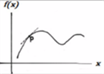Neither f'(a) < 0 Negative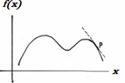Neither f'(a) = 0 Zero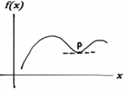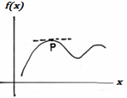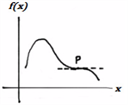Local Minimum       Local Maximum         Neither Not Defined Not Defined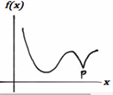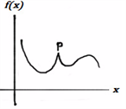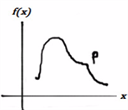Local Minimum       Local Maximum       Neither

### What is Critical Point?

In mathematics, a Critical point of a differential function of a real or complex variable is any value in its domain where its derivative is 0. We can hence infer from here that every local extremum is a critical point but every critical point need not be a local extremum. So, if we have a function which is continuous, it must have maxima and minima or local extrema. This means that every such function will have critical points. In case the given function is monotonic, the maximum and minimum values lie at the endpoints of the domain of the definition of that particular function.

Maxima and minima are hence very important concepts in the calculus of variations, which helps to find the extreme values of a function. You can use these two values and where they occur for a function using the first derivative method or the second derivative method.

To know and learn new concepts every day, download BYJU’S – The Learning App.